# Add a formula to a search

Learn how to add a formula to a search.

You can add a formula directly within a search. Some common reasons for using a formula in a search are to perform mathematical functions, check for and replace null values, or add conditional logic.

To create a formula in a search, follow these steps:

1. Start a new search, or choose an existing answer from a pinboard to edit.

2. If the answer shows a chart, switch to Table View.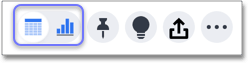3. In the upper-right side of the table, click the more options icon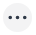and select Add formula.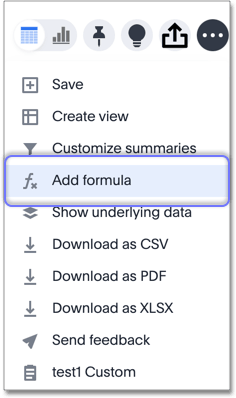4. Name and enter your formula in the Formula Builder.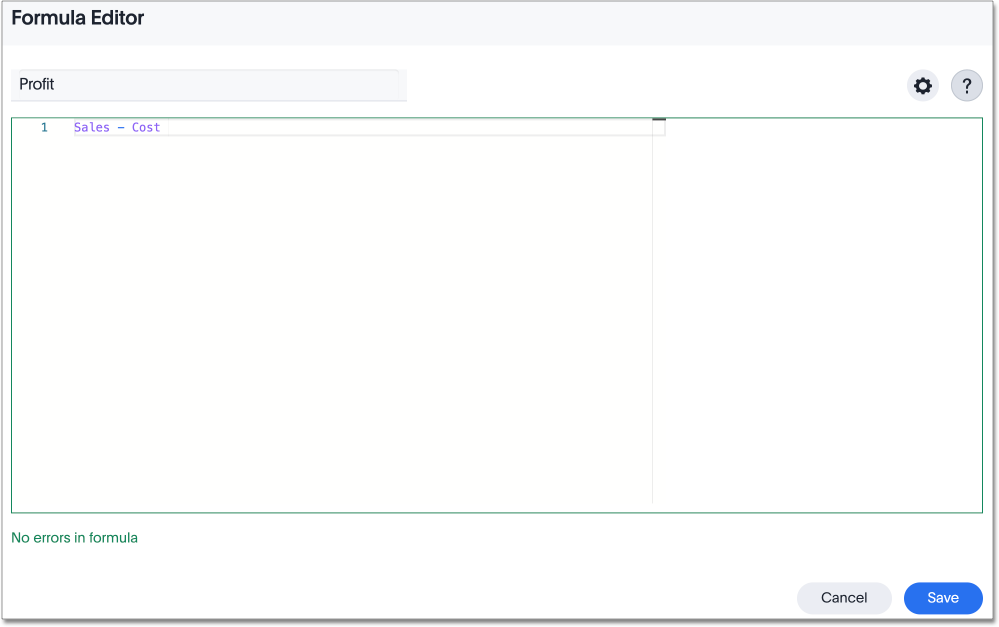Formula elements are color coded by type and can include the formula operators and functions (blue), the names of columns (purple), and/or constants (black).
5. You can see a list of formula operators with examples by clicking Formula Assistant. Expand a category, such as Operators, and click on a formula operator for a definition and example.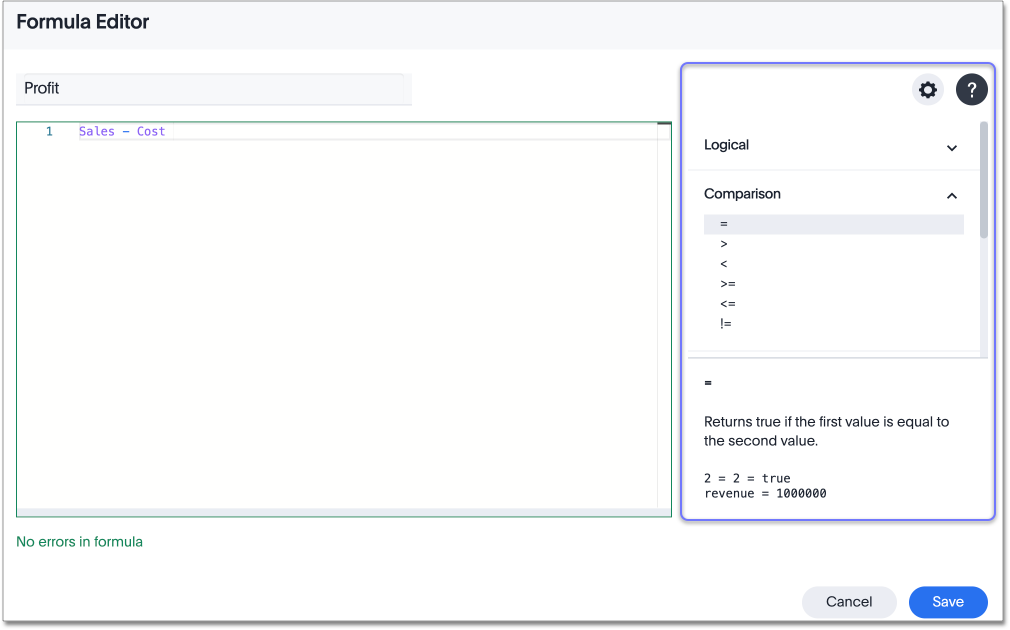6. If you want to change what your formula returns, use the Advanced settings. Depending on your formula, you may be able to change the following aspects:

• Data type: Determines the type of data that the formula generates. For example, use `date` for an `add_days` formula.

• Measure or attribute: Determines if the output of the formula is a measure or an attribute. For example, choose `attribute` for a formula that generates age groups, and choose `measure` for a formula that generates profit.

• Aggregation: Determines the default aggregation of the formula output. For example, choose `min` to see minimum profit.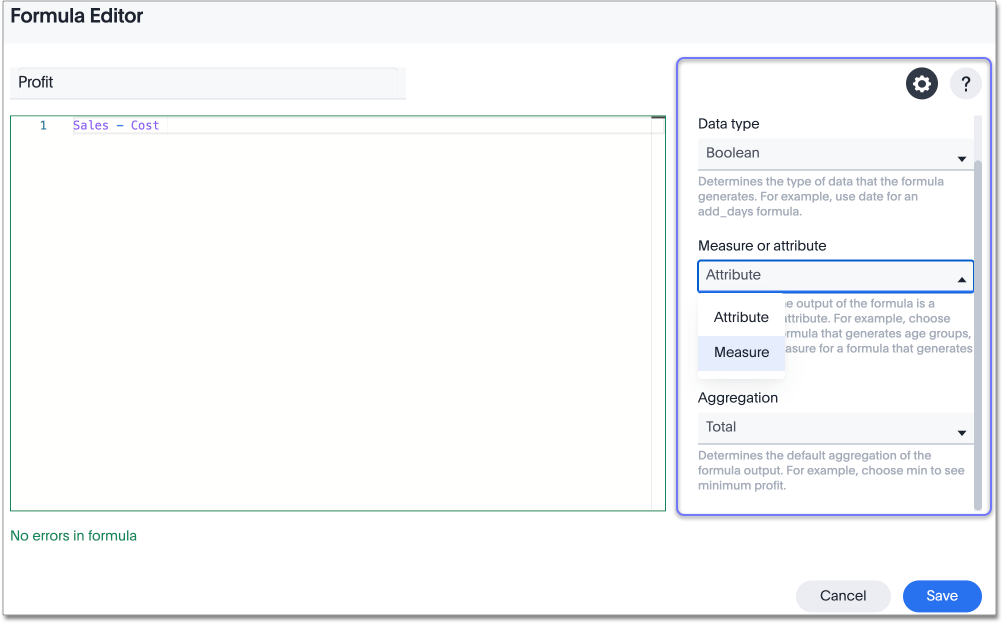7. Click Save. Name your formula, if you did not already name it.

Related information